# Running Operations for Vectors

Fully supports rolling windows operations by controlling window length, window lag, time indexing. With runner one can apply any R function on rolling windows. Package eases work with equally and unequally spaced time series.

# sportPackage contains standard running functions (aka. windowed, rolling, cumulative) with additional options. `runner` provides extended functionality like date windows, handling missings and varying window size. `runner` brings also rolling streak and rollin which, what extends beyond range of functions already implemented in R packages.

## Installation

Install package from from github or from CRAN.

## Examples

The main idea of the package is to provide running operations on R vectors. Running functions are these which are applied to all elements up to actual one. For example implemented already in `base` `cumsum`, `cummin` etc. Functions provided in this package works similar but with extended functionality such as handling `NA` and custom window size. The most functions provided in package are based on the same logic:

• window size `k` denotes number of elements from i-th backwards, where functions are calculated. `k` can be also specified by vector of the same length as x as illustrated in second example.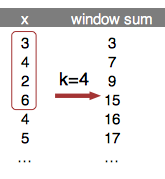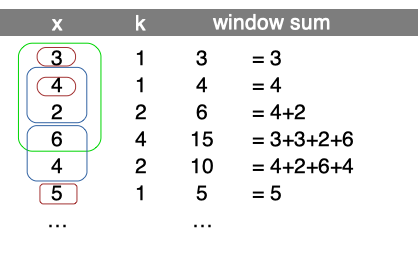• argument `na_rm=T` handling missing and is equivalent to `na.rm`.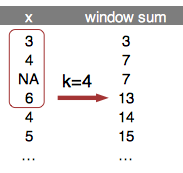• `na_pad=T` if window size exceeds number of available elements, than first `k-1` elements are filled with `NA`.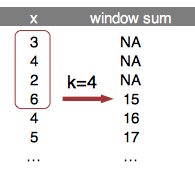• `which` used with running index, which value ('first' or 'last')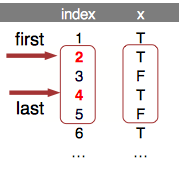• `idx` to calculate within time window. `idx` is a date (or numeric) vector of the same length as x. `k` then should to be an integer specyfying time window span. In the example k=5 window sum.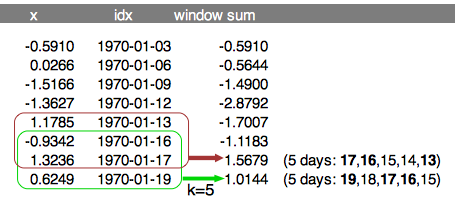### Creating windows

Function creates list of windows. Because `runner` provide limited functionality, one can create running-window-list which can be further processed by user to obtain desired statistic (eg. window sum). `x` is a vector to be 'run on' and `k` is a length of window. In this example window length is varying as specified by `k`. Provide one value to obtain constant window size.

Such windows can be used in further calculations, with any R function. Example below shows how to obtain running `sum` in specified, varying window length (specified by `k`).

One can also specify window based on a date of other numeric index. To do this date should be passed via `idx` argument and k should be integer denoting date-window span.

### Unique elements in window

User can use `unique_run` create list of unique elements within specified window size.

### Running aggregations `(mean|sum|min|max)_run`

Runner provides basic aggregation methods calculated within running windows. Below example showing some functions behavior for different arguments setup. Let's take a look at 8th element of a vector on which `min_run` is calculated. First setup uses default values, so algorithm is looking for minimum value in all elements before actual (i=8). By default missing values are removed before calculations by argument `na_rm=TRUE`, and also window is not specified. The default is equivalent of `base::cummin` with additional option to ignore `NA` values. In second example within window k=5, the lowest value is -3. In the last example minimum is not available due to existence of `NA`. Graphical example is reproduced below in the code.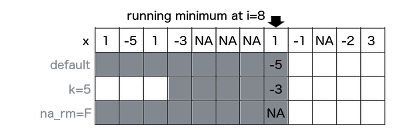### fill_run

Function used to replace `NA` with previous non-NA element. To understand how `fill_run` works, take a look on illustration. Row 'x' represents, and another rows represent replaced `NA` by fill_run with different options setup (run_for_first=TRUE and only_within=TRUE respectively). By default, `fill_run` replaces all `NA` if they were preceded by any value. If `NA` appeared in the beginning of the vector then it would not be replaced. But if user specify `run_for_first=TRUE` initial empty values values will be replaced by next non-empty value. Option `only_within=TRUE` means that `NA` values would be replaced if they were surrounded by pair of identical values. No windows provided in this functionality.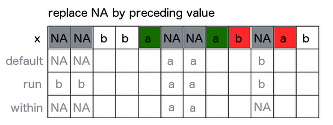### Running streak

To count consecutive elements in specified window one can use `streak_run`. Following figure illustrates how streak is calculated with three different options setup for 9th element of the input vector `x`. First shows default configuration, with full window and `na_rm=T`. Second example count within k=4 window with count reset on `NA`. Last example counting streak with continuation after `NA`. Visualization also supported with corresponding R code.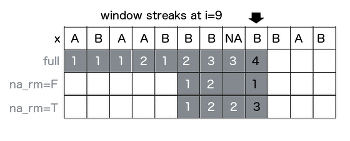### Running which

To obtain index number of element satisfying some condition in window, one can use running which. Functions from this group returns index while condition is `TRUE` appeared before n-th element of a vector. In below example `whicht_run` function returns index of element containing `TRUE` value. If `na_rm=T` is specified, missing is treated as `FALSE`, and is ignored while searching for `TRUE`. While user set `na_rm=F` like in second example, function returns `NA`, because in following window `TRUE` appears after missing and it's impossible to be certain which is first (missing is an element of unknown value - could be TRUE or FALSE).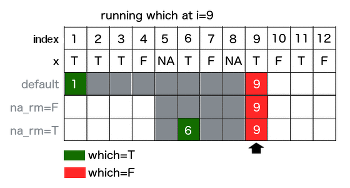# runner 0.2.0

## Improvements

• all functions have additional `idx` argument which allows to compute running windows within specified date/time/indexes range.
• added lag_run function - lagged value able to be computed on date/time lag.
• added length_run function to compute number of elements within specified date/time/indexes range.

# Reference manual

install.packages("runner")

0.3.7 by Dawid Kałędkowski, 2 months ago

Report a bug at https://github.com/gogonzo/runner/issues

Browse source code at https://github.com/cran/runner

Authors: Dawid Kałędkowski [aut, cre]

Documentation:   PDF Manual